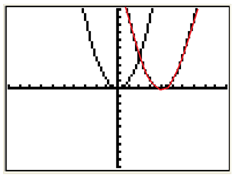Equations

When writing the equation of a transformation, determine how the graph has moved in the coordinate plane compared to the parent function and then use the rules to write an equation.

Example 1 The graph of y = x2 is transformed to produce the new red graph. What's the new equation?Step 1. Determine the transformation of the graph.

This graph has been translated 4 units right.

Step 2. Use the transformation rules to write an equation.

f(x - b) translates the graph to the right.

The equation will be:
y
= (x - 4) 2